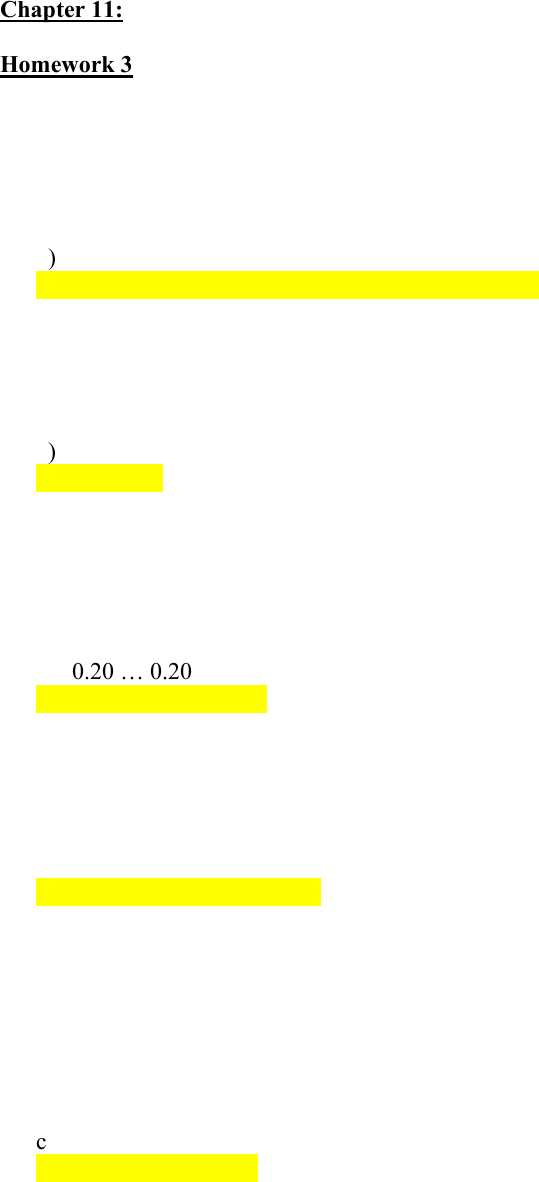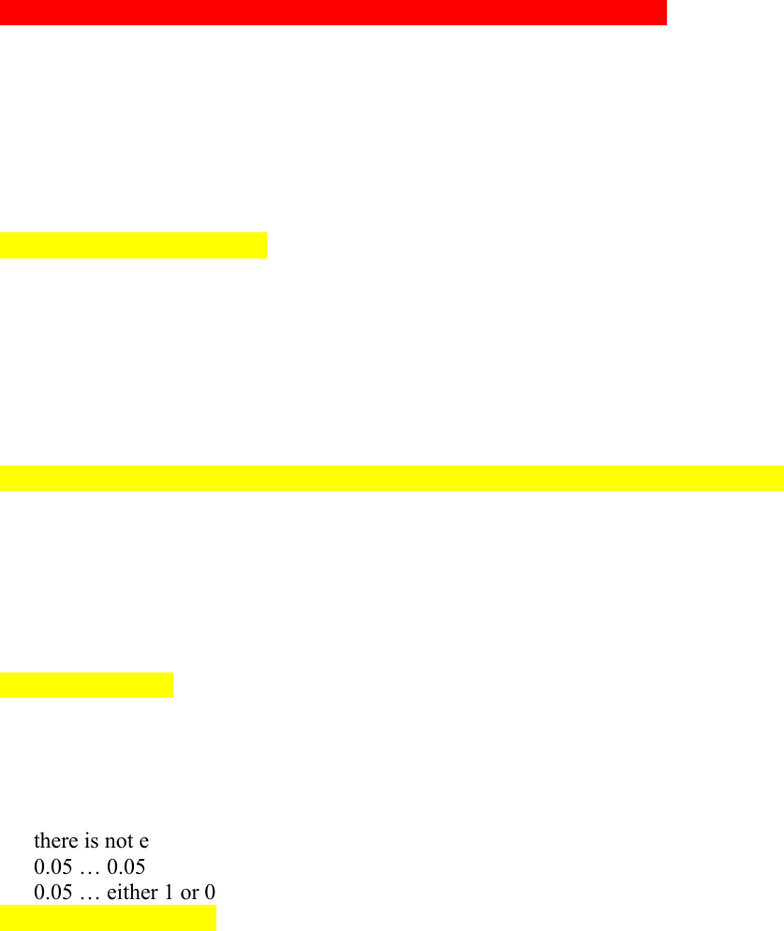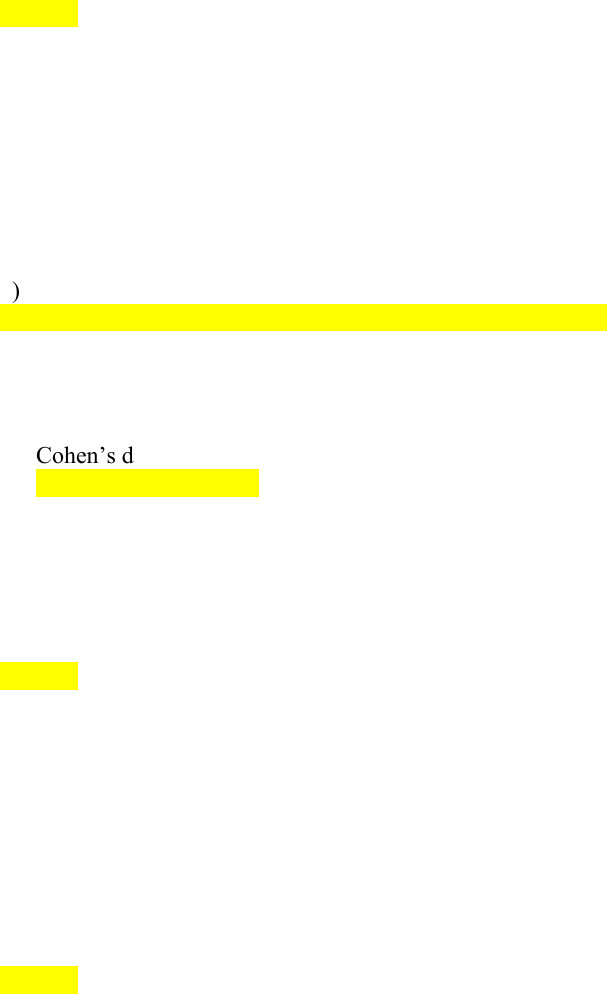# PNB 3XE3 Study Guide - Quiz Guide: Standard Deviation, Type I And Type Ii Errors, Pooled Variance

74 views8 pages
School
McMaster University
Department
Psychology, Neuroscience & Behaviour
Course
PNB 3XE3Chapter 11:
Homework 3
1. If you are comparing two groups, what of the following statistic will you NOT
include in your calculation of Cohen’s d? (Assume equal standard deviations across
groups)
a) the mean of the control group
b) the standard deviation of the control group
c) the standard deviation of the experimental group
d) the mean of the experimental group
2. In order to claim that you have a large effect, your Cohen’s d should be:
a) at least .2
b) at least .5
c) at least .8
d) at least .99
3. Once completed, and assuming beta = 0.20, the probability of a type II error on an
individual test is __, and the probability of a type II error across 1000 identical tests
is __.
a) 0.20 0.20
b) either 1 or 0 … 0.20
c) 0.20 … either 1 or 0
d) there is not enough information to answer this question
4. You conduct a significance test across a huge sample. The resulting p value is less
than 0.05. This likely means that:
d) all of the above
5. What are the units of measurement of a z score?
a standard errors of the mean
b means
c variance
d standard deviations
Unlock document

This preview shows pages 1-3 of the document.
Unlock all 8 pages and 3 million more documents.6. Which of the following is true?
a) the effect size r = 0.30 is three times as big as the effect size r = 0.10
b) the effect size r = 0.50 is twenty times as big as the effect size r = 0.10
c) the effect size r = 0.80 is twice as big as the effect size r = 0.40
d) the effect size r = 0.80 is nine times as big as the effect size r = 0.40
7. Increasing your sample size will make it more likely that:
c) you can accept the null hypothesis
8. How is it that intentions of scientists influence NHST?
a) people are biased and will, consciously or unconsciously, interpret the results as
consistent with their expectations
b) the number of participants who actually participated in the experiment should determine
the p-value, regardless of the number of participants that the experimenter intends to
collect from
c) p value that is appropriate depends on what your intentions were for collecting data
d) all of the above
9. An example of effect size is
a) Cohen’s d
b) Pearson’s correlation
c) the odds ratio
d) all of the above
10. Once completed, and assuming alpha = 0.05, the probability of a type I error on an
individual test is __, and the probability of a type II error across 1000 identical tests
is __
a) there is not enough information to answer this question
b) 0.05 0.05
c) 0.05 either 1 or 0
d) either 1 or 0 … 0.05
Unlock document

This preview shows pages 1-3 of the document.
Unlock all 8 pages and 3 million more documents.11. Given the following table, what are the odds of hearts remaining? (figure 11.1 page
379)
a) 1.56
b) 2.56
c) 4
d) 25
12. In order to quantify the effect size of an experiment, you compare the mean of one
group to the mean of the other group. All of the following will make this effect size
hard to compare except:
a) the mean will be expressed in the units of this study’s measurements
b) this figure accounts for the signal, but not the noise
c) the construct that is being measured is specific to this study
d) none of the above
13. Which of the following has a maximum of 1?
a) Cohen’s d
b) Pearson’s correlation, r
c) the odds ratio
d) all of the above
14. Imagine you are comparing two groups. A group of 20 participants has a standard
deviation of 10, and a group of 10 participants has a standard deviation of 2. What
is the pooled standard deviation?
a) 8.32
b) 53.58
c) 8.13
d) 7.14
e) there isn’t enough information to make this calculation
15. Imagine the mean of your control group (n = 1000) was 10 and the standard
deviation 6. The mean of your experimental group (n = 10) was 15 and the standard
deviation 10. Compute Cohen’s d.
a) 0.625
b) 0.83
c) 0.5
d) 1.5
e) There is not enough information to make this calculation
Unlock document

This preview shows pages 1-3 of the document.
Unlock all 8 pages and 3 million more documents.